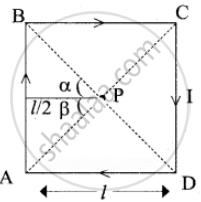Tamil Nadu Board of Secondary EducationHSC Science Class 12th

# Calculate the magnetic field at the center of a square loop which carries a current of 1.5 A, length of each loop is 50 cm. - Physics

Numerical

Calculate the magnetic field at the center of a square loop which carries a current of 1.5 A, length of each loop is 50 cm.

#### SolutionCurrent through the square loop, I = 1.5A

Length of each loop, l = 50cm 50 x 10-2 m

According to Biot-Savart Law.

Magnetic field due to a current-carrying straight wire

B = (mu_0"I")/(4pi"a") (sin alpha + sin beta)

= (4pi xx 10^-7 xx 1.5)/(4pi xx (l/2)) (sin45^circ + sin 45^circ)

= (2 xx 1.5 xx 10^-7)/"l"(1/sqrt2 + 1/sqrt2)

= (2 xx 1.5xx10^-7)/(50xx10^-2)(2/sqrt2)

B =0.084866 x 10-5 T

Magnetic field at a point p? of centre of current carrying square loop

B’ =4 sides x B

= 4 x 0.08487 x 10-5 = 0.33948 x 10-5

B’ =3.4 x 10-6 T

Concept: Magnetic Effects of Current
Is there an error in this question or solution?

#### APPEARS IN

Tamil Nadu Board Samacheer Kalvi Class 12th Physics Volume 1 and 2 Answers Guide
Chapter 3 Magnetism and magnetic effects of electric current
Evaluation | Q IV. 6. | Page 193
Share